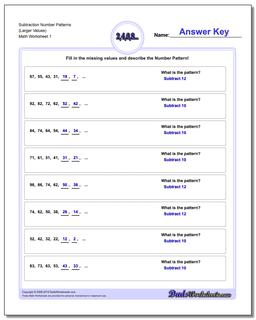# Math Worksheets: Number Patterns: Number Patterns: Subtraction Number Patterns (Larger Values)## Subtraction Number Patterns (Larger Values)

PropertyValue
DescriptionSubtraction Number Patterns (Larger Values): Number pattern problems using only subtraction operations.
Resource TypeWorksheet